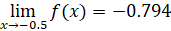It looks like you're using Internet Explorer 11 or older. This website works best with modern browsers such as the latest versions of Chrome, Firefox, Safari, and Edge. If you continue with this browser, you may see unexpected results.

# Math

Some of the content of this guide was modeled after a guide originally created by the Openstax and has been adapted for the GPRC Learning Commons in September 2021. The graphs are generated using Desmos. This work is licensed under a Creative Commons BY 4.0 International License.## Limit

The limit of a function can be generalized as:

"as the value of x approaches a, f(x) approaches L."

The formula is written as shown below, where L is any real number and where x approaches but does not equal :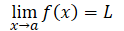## Functional Values

One method to find a limit of a function is to use the values of nearby variables and observe how the result of the function trends towards a common value from the lower and higher variables.  The table below shows the limit of f(x)=x² as x approaches 2 and is an example of evaluating a limit using functional values.

x f(x) = x² x f(x)=x²
1.0 1.0 3.0 9.0
1.5 2.25 2.5

6.25

1.75 3.063 2.25 5.063
1.875 3.516 2.125 4.516
1.95 3.802 2.05 4.202
1.99 3.96 2.01 4.04
1.999 3.996 2.001 4.004

Table 1: x approaching 2 for f(x)=x²

As the value of x gets closer and closer to 2, the value of f(x) gets closer and closer to 4.  From this result, we can write the notation below: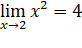## Basic Limits

Basic limits are true for any real number a and any constant c.
Property Description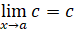The limit of a constant, c, is equal to c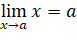The limit of the variable x as it approaches a is equal to a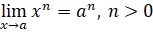The limit of the variable x raised to the power n is the value of a raised to the power n

• Where n is greater than zero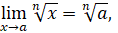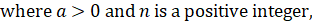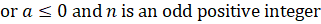The limit of the variable x to the root of n is the value of a to the root of n

• Where a is greater than zero and n is a positive integer

or

• Where a is less than or equal to zero and n is an odd positive integer

Table 2: Basic Limits Results

## One-Sided Limits

Not all functions can be defined at all points, so there is a concept of one-sided limits.  One-sided limits can be described as:

• "approaching from the left" by only viewing the functional values for x < a with the form,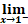or

• "approaching from the right" by only viewing the functional values for x > a with the form,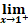The function shown below has an undefined value at x = 1 or x = –1 and as we will show, two separate limits from the left and the right for x = 1.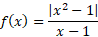The function can be rewritten as a combination of two functions over a specific domain: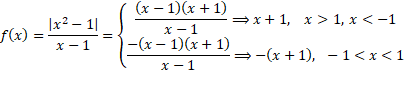With the new equations for the function, we can find the limits from the left, where x < 1, and the right, where x > 1: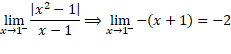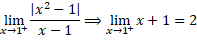Although we are able to determine a left sided limit and a right sided limit, these two limits are not equal.  As there is no single number that the function approaches, we can conclude that the limit of the function does not exist.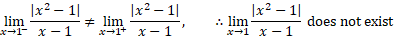## Examples

Example 1: The limit of a constant
For the plot shown below, we are viewing the function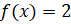As x approaches the value 4, we can see visually that the function will trend towards the value 2 from both the left and the right.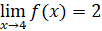Example 2: The limit of a variable

For the plot shown below, we are viewing the function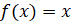As x approaches the value 4, we can see visually that the function will trend towards the value 4 from both the left and the right.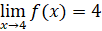Example 3: The limit of a power

For the plot shown below, we are viewing the function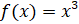As x approaches the value 0.5, we can see visually that the function will trend towards the value 0.125 from both the left and the right.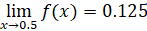Example 4: The limit of a positive value and an even root

For the plot shown below, we are viewing the function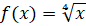As x approaches the value 0.5, we can see visually that the function will trend towards the value 0.841 from both the left and the right.  As this is an even root, the function is not valid for negative values of x.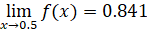Example 5: The limit of a negative value and an odd root

For the plot shown below, we are viewing the function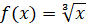As x approaches the value –0.5, we can see visually that the function will trend towards the value – 0.794 from both the left and the right.  As this is an odd root, the function is valid for negative values of x.